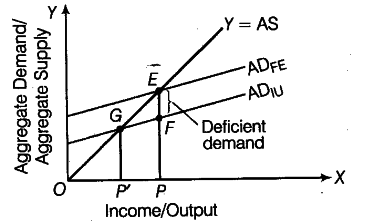# Explain the concept of deficient demand

Explain the concept of deficient demand in macroeconomics. Also explain the role of bank rate in correcting it.

A situation in an economy, when the Aggregate Demand is less than the Aggregate Supply, corresponding to full employment level, is termed as deficient demand.
In the following figure, E is the equilibrium point of the economy where AD = AS. But at the current deficient demand of {{AD}_{IU}}, Aggregate Demand FP is less than the Aggregate Supply of, EP. Hence, EF represents the deficient demand in the economy.
Deficient demand gives rise to a deflationary gap and leads the economy to an equilibrium level of income/output that is less than the full employment level of income. This leads to deflationary pressures on economy and increases the inventory of producers. The producers are discouraged to produce more as price levels fall.
The economy therefore, will attain a new equilibrium at point G with National Income of OP’.